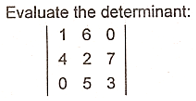# Solution: Find the determinant of the given 3 x 3 matrix.

(Last Updated On: March 28, 2019)#### Problem Statement: EE Board April 1997

Evaluate the determinant of the 3 x 3 matrix.#### Problem Answer:

The determinant of the 3 x 3 matrix is equivalent to -101.

### Latest Problem Solving in Advanced Engineering Mathematics

More Questions in: Advanced Engineering Mathematics

#### Online Questions and Answers in Advanced Engineering Math

Solution: Find the determinant of the given 3 x 3 matrix.
5 (100%) 1 vote[s]

NEED SUPPORT: LIKE MY PAGE & SHARE